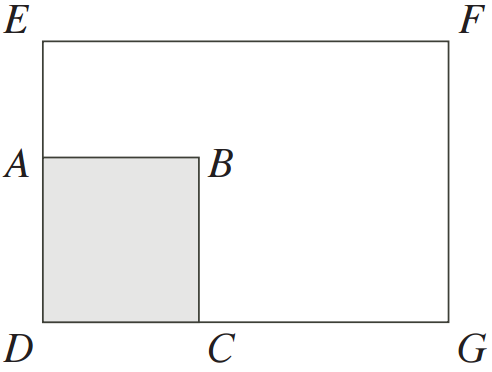# The Case Of The Missing Square

Geometry Level 1

Rectangle $DEFG$ has square $ABCD$ removed leaving an area of $92\text{ m}^2$. Side $AE = 4\text{ m}$ and side $CG = 8\text{ m}$.

Determine the original area (in $\text{m}^2$) of rectangle $DEFG$.×